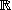# OEF subspace definition --- Introduction ---

This module actually contains 21 exercises on the definition of subspaces of vector spaces. You are given a vector space and a subset defined in various ways; up to you to determine whether the subset is a subspace.

See also the collections of exercises on vector spaces in general or defition of vector spaces.

### Continuous functions

Let be the -vector space of real functions

,

and let be the subset of composed of the function 0 and functions which are on [,].

Is a subspace of ?

### Increasing functions

Let be the -vector space of real functions

,

and let be the subset of composed of the function 0 and functions which are on [,].

Is a subspace of ?

### Crossed Matrices

Let be the vector space of matrices of size , and let be the subset of composed of matrices such that

Is a subspace of ?

### Matrices and determinant

Let be the vector space of matrices of size ×, and let be the subset of composed of matrices M whose is equal to .

Is a subspace of ?

### Matrices and elements

Let be the vector space of matrices of size ×, and let be the subset of composed of matrices M such that .

Is a subspace of ?

### Multiplied matrices

Let be the vector space of matrices of size , and let be the subset of composed of matrices
.

Is a subspace of ?

### Matrices and rank

Let be the vector space of matrices of size ×, and let be the subset of composed of matrices of rank .

Is a subspace of ?

You must give all the good replies.

### Matrices with power

Let be the vector space of matrices of size ×, and let be the subset of composed of matrices M such that M=0.

Is a subspace of ?

### Periodic functions

Let be the vector space of real continuous functions over , and let be the subset of composed of the function 0 and periodic functions whose period .

Is a subspace of ?

### Polynomials and coefficients

Let be the -vector space of real polynomials P(X) of degree less than or equal to , and let be the subset of composed of polynomials such that the of its coefficients equals .

Is a subspace of ?

### Polynomials and degrees

Let be the vector space of polynomials over , and let be the subset of composed of polynomials with degree .

Is a subspace of ?

### Polynomials and integral

Let be the vector space over of polynomials, and let be the subset of composed of polynomials such that

Is a subspace of ?

### Polynomials and integral II

Let be the vector space over of polynomials, and let be the subset of composed of polynomials such that

Is a subspace of ?

### Polynomials and roots

Let be the -vector space of real polynomials P(X) of degree less than or equal to , and let be the subset of composed of polynomials such that the of its roots (real or complex, counted with multiplicity) equals 0.

Is a subspace of ?

### Polynomials and roots II

Let be the -vector space of real polynomials P(X) of degree less than or equal to , and let be the subset of composed of polynomials with .

Is a subspace of ?

### Polynomials and values

Let be the vector space of polynomials over , and let be the subset of composed of polynomials such that .

Is a subspace of ?

### Polynomials and values II

Let be the vector space of polynomials over , and let be the subset of composed of polynomials (X) such that .

Is a subspace of ?

### Polynomials and values III

Let =[X] be the vector space of polynomials over , and let be the subset of composed of polynomials such that .

Is a subspace of ?

### Real functions

Let be the -vector space of real functions

,

and let be the subset of composed of functions (x) .

Is a subspace of ?

### Square matrices

Let be the vector space of matrices of size ×, and let be the subset of composed of matrices.

Is a subspace of ?

### Vectors of R^3

Let be the vector space of dimension 3 over , and let be the subset of composed of vectors such that .

Is a subspace of ?

Other exercises on: vector spaces   linear algebra

The most recent version

This page is not in its usual appearance because WIMS is unable to recognize your web browser.

In order to access WIMS services, you need a browser supporting forms. In order to test the browser you are using, please type the word wims here: and press Enter''.

Please take note that WIMS pages are interactively generated; they are not ordinary HTML files. They must be used interactively ONLINE. It is useless for you to gather them through a robot program.

Description: collection of exercices on the definition of subspace of vector spaces. interactive exercises, online calculators and plotters, mathematical recreation and games

Keywords: interactive mathematics, interactive math, server side interactivity, algebra, linear algebra, vector space, subspace, polynomial, matrix, function